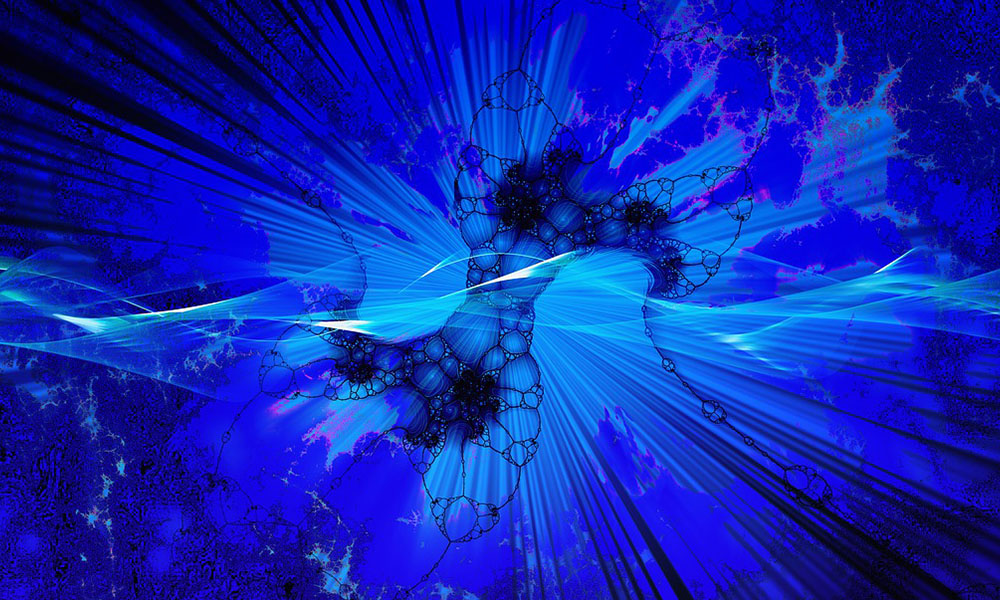# A String Theory for Particle Quanta

In my book on aether physics, I propose that inertial matter is created from photons through electron-positron pair production, a process that transforms photons into electron-positron pairs. Thus, a single compact particle expands into two bloated nets.

This suggests that particle quanta are made up of strings that are either tightly bound or inflated into nets. In their tightly bound state, they connect together to form neutrinos and photons. In their inflated state, they form electrons and positrons which in turn combine to produce atoms with atomic nuclei and associated electrons.

If we choose to go with this model, we can further deduce the makeup of particle quanta. With only three types of such quanta, we know that each particle quantum has to be constituted of exactly two strings. Neutral quanta, which are an equal mix of positive and negative, are bundles of one positive and one negative string. Positive quanta consist of two positive strings, and negative quanta consist of two negative strings.

From this, it is clear that it is the strings that give particle quanta their texture. Positive strings have an abrasive texture, and negative strings have a woolly texture. If we further assume that the assembly of particle quanta are completely random, we can conclude that there must be two neutral particle quanta for every positive and negative quantum pair. This follows from a simple consideration of all possible combinations:

• negative + negative string = negative particle quantum
• positive + positive string = positive particle quantum
• positive + negative string = neutral particle quantum (neutrino)
• negative + positive string = neutral particle quantum (neutrino)

Since positive and negative particle quanta only exist as part of some larger assembly, we get that the number of neutrinos in the aether must be at least 6 times more than photons. This is because every photon consists of 3 positive and 3 negative particle quanta. For every photon there are six free neutral particle quanta.

However, the relative number of neutrinos are even larger because many photons are converted into inertial matter through electron-positron production. With a lot of photons tied up in inertial matter, there are correspondingly fewer photons to make up the aether.

### This Post Has 0 Comments

This site uses Akismet to reduce spam. Learn how your comment data is processed.